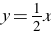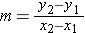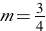# Slope-Intercept

You have learned what makes a relation a function. Now, it is time to look at a particular function called a linear function. The word linear has the word line in it, so it’s easy to remember what shape a linear function is….it is a line!

In previous years, you’ve studied proportions. This school year, you’ve learned about slope. Now it is time to combine these two pieces of content. We can represent a proportional relationship by a linear graph where the proportion is the slope of the line and the y -intercept is at zero.

Recall that slope-intercept form is described as y = mx + b       where x and y represent coordinates, m represents slope, and b represents the y-intercept.

When we are looking at a linear graph whose y-intercept is 0, then our equation would be y = mx + 0, or just y = mx. This indicates that there is a direct proportion between x and y.

Let’s look at an example:

John’s dad owns a car dealership. John helps out by washing cars on Saturdays. John earns \$10 in iTunes gift cards for every 2 cars he washes. So, the proportion of gift cards earned to the number of cars washed is 10 to 2. Of course, we can reduce that to 5 to 1.

The amount in gift cards earned for x number of cars washed can be represented by a line. The slope of that line is the proportion represented by the above ratio, which written as a fraction is 5/1, or 5, and the y-intercept is zero.

We can represent this with the following equation: y = 5x

A graph of this equation is shown below.We discussed in our unit on functions that slope and rate of change can be used synonymously. In addition, we learned that the rate of change is determined by the change in the y values divided by the change in the x values. This is represented with the following formula:

So, what is our unit rate on this example? Let’s pick two points and use the definition of rate of change to determine the rate of change. We’ll use the points (0, 0) and (5, 1); however, any two points on the line will yield the same result since it is a linear function, i.e., a line.Therefore, the rate per gift card amount is \$5 per car washed.

We could have also stated that since we know we are looking for unit rate, we can use the formula y=kx which shows k as the constant of proportionality. To solve for k, the constant of proportionality, or the unit rate, we can say k = y/x = 10/2 = 5. Either way, the unit 5, or slope is \$5/car.

As we have seen in the above discussion and example, a proportional relationship can be represented by the equation y = mx where m is the slope or rate of change. Remember on these examples our y-intercept is zero, which means the line passes through the origin. Having b as 0 just means that the relationship that exists between x and y is directly proportional, and our m, or slope, is our constant of proportionality.

We can compare the rates of change between equations. The equation with the greater value of m, or slope, will have the greater rate of change.

Example:
Compare the rates of change of the following equation and table. Determine which has the greater rate of change.

x y
0 0
4 3
8 6
12 9

First, examine the slope, or rate of change, for the graph and for the table separately. Then, compare the two slopes to determine which is larger.

For the equation,, the rate of change can be pulled directly from the equation. Because it is already in slope-intercept form, y = mx + b, we can see directly that the coefficient of x, labeled as m, is the slope, or rate of change, of the equation.

Therefore, the rate of change for the equation is ½.

Now, let’s take a look at the table. Here, it is easiest to use the formula for finding the rate of change. The formulatells us how to find slope. We can pick any two points from the table and substitute them into this formula.

(4, 3) and (12, 9) will be the points we’ll substitute into the formula.Therefore, the rate of change of the table is ¾. Please keep in mind that we would have gotten ¾ for any two points chosen.

Since ¾ > ½, the rate of change of the table is greater than that of the equation.

Notice that we can use any two points to determine the slope of the line defined by the points in the table. Why is this?

The slope of a line is the same between any two pairs of points on the line. This can be demonstrated using similar triangles.

(edited from: source)